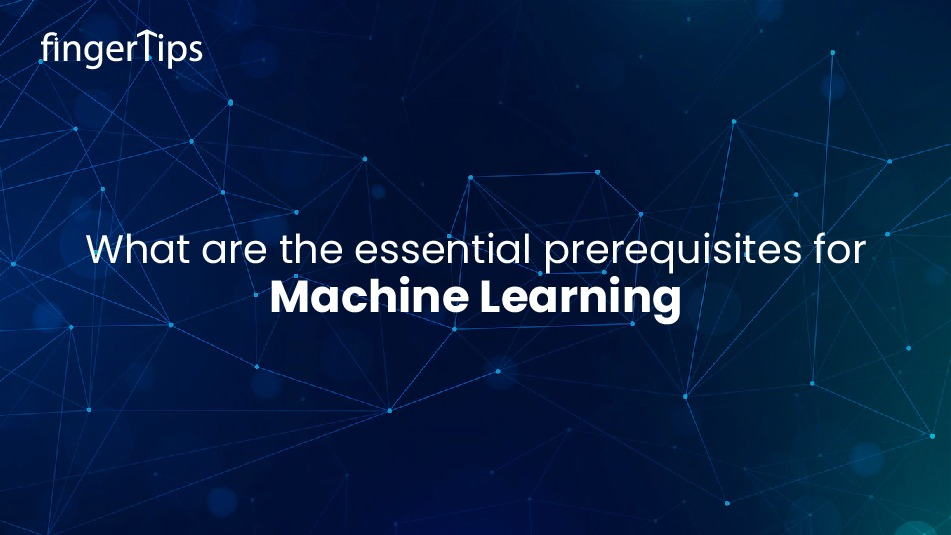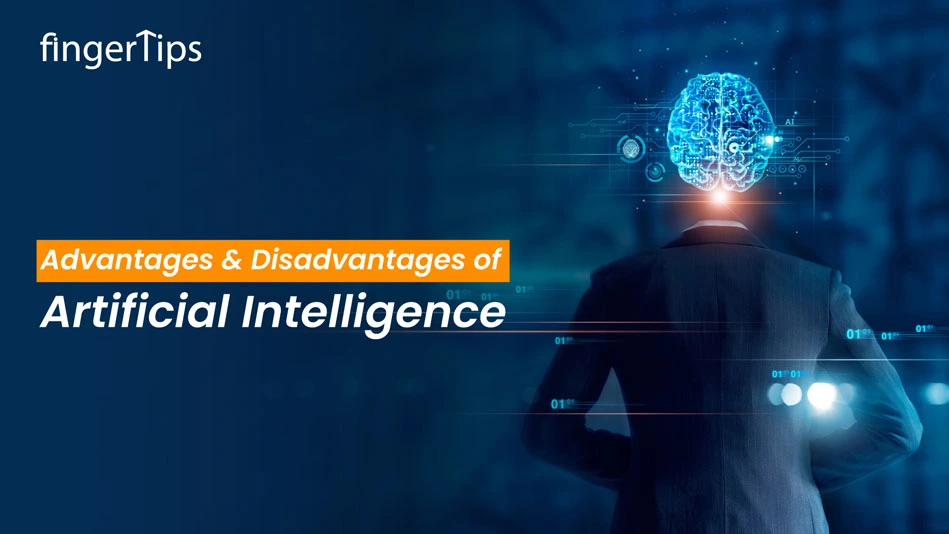Home > Blog > Artificial Intelligence > What Are The Essential Prerequisites For Machine Learning?

# What Are The Essential Prerequisites For Machine Learning?

21 Dec 2022
569### Related TopicsInterested in this course?Machine Learning is the most in-demand technology today. Are you a fresher and want to explore the Machine Learning world? You must be familiar with the prerequisites for Machine Learning. This article will help you understand the many concepts you need to know before you begin the exciting journey with Machine Learning.

Here are some of the machine learning prerequisites to get you started.

## Prerequisites to Learn Machine Learning

• Statistics
• Probability
• Linear Algebra
• Calculus

### Statistics

What are statistics? It is a complete control system, mainly dealing with data collection, sorting, analysis, translation, and presentation with reports and charts. It is undoubtedly the most valuable feature of machine learning, and data holds immense importance regarding technology.

It Lets us understand how statistics define a vital aspect of machine learning.

Machine learning comes in two different types, and one is descriptive statistics and second is inferential statistics.

As the name reflects, descriptive statistics deals with the numbers that describe different types of data sets and models. It concludes the available data sets and extracts them into meaningful information. On the other hand, inferential statistics deviate from the part of the data instead of the entire data set or model.

A machine learning professional will have to be familiar with the following:

• Mean
• Median
• Standard deviation
• Outliers
• Probability

Probability is defining a possibility as how likely any action of the plan is about to happen. Probability is the system's main base and foundation, which anticipates important data-driven decisions.

In machine learning, the following concepts are essential for aspiring machine learning students will be working with.

• Notation
• Rules of probability, Bayes theorem, product rule, and sum rule
• Independence
• Continuous random variables
• Probability distribution, joint and conditional

### Linear Algebra

Linear algebra is an essential part of a machine learning algorithm, and the relationship between the two is unique. The matrix operations and the complex concepts make it a little abstract to deal with the procedures. Linear algebra, in machine learning, deals with the following concepts:

• Notations
• Matrix multiplication
• Tensor and tensor rank
• Coding Algorithms
• Linear transforms

### Calculus

Calculus is the most compelling aspect of building a machine-learning model. With a better understanding of calculus, you may achieve your target of excelling in machine learning. You must be efficient in the following points.

• General knowledge of integration
• Partial derivatives
• Chain rule—for training neural networks
• Programming Languages

Having prior knowledge of programming language adds excellent effect when adopting machine learning expertise. Even though it is more of a mathematical front, having profound knowledge of any one programming language will constantly add more advantages to your learning process of knowing machine learning well. Selecting the programming language that can be implemented in the machine learning algorithms will be an added value to your motive.

Some of the popular programming languages:

### Python

Python's smooth syntax, built-in functions, and comprehensive package adds more value and make it famous for machine learning, especially among keen learners. It has a fantastic collection of supportive and helpful libraries. The Python Package Index (PyPI) allows you to access approx. 235,000 packages.

Python covers the following topics:

• NumPy for mathematical operations
• Scikit-learn for algorithm regression and classification
• pandas for file operations
• Matplotlib for data visualization
• TensorFlow for Deep Learning
• PyTorch for deep learning
• OpenCV and Dlib for computer vision

### R

R is one of the most significant AI and machine learning prerequisites that Python majorly uses. Many crucial machine learning algorithm applications are arrayed through R. It has good library support and graphs.

Following are the packages that are supported by R:

• Kernlab and Caret support the operations that deal with the   regression and classification
• Rpart and SuperML for machine learning
• Mlr3 for machine learning workflows
• Plotly and ggplot for data visualization
• DataExplorer for data exploration
• Unlike Python, R is comparatively slower than C++ and can be difficult for learners.

MATLAB

MATLAB has a set of predefined functions along with a graphical user interface. MATLAB is a no-syntax program assigned to share programs as independent apps and web applications. MATLAB profoundly supports machine learning. MATLAB provides optimized and reduced code sets through AutoML. Automated code generation provides a sensor analytics function.

MATLAB is loaded with positive prospects but is not a free tool. Therefore a lot of the MATLAB community is from the research field.

## Conclusion

It is essential to know about the prerequisites for machine learning; it is also important to understand how to work with data. It is a highly recommended skill that one must pursue to master machine learning algorithms. This article explored the factors considered to know the prerequisites of machine learning, along with some preferred programming languages. The machine learning algorithm is a set of functionalities that includes statistics, calculus, linear algebra, probability, and programming languages.

Get yourself trained or upskilled with the associate factors to be a skillful machine learning professional. Understand your forte and get started!Fingertips